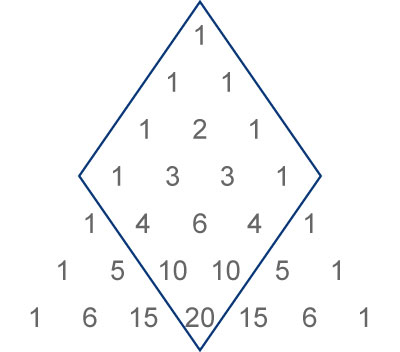# pascal

## Syntax

``P = pascal(n)``
``P = pascal(n,1)``
``P = pascal(n,2)``
``P = pascal(___,classname)``

## Description

example

````P = pascal(n)` returns a Pascal’s Matrix of order `n`. `P` is a symmetric positive definite matrix with integer entries taken from Pascal's triangle. The inverse of `P` has integer entries.```
````P = pascal(n,1)` returns the lower triangular Cholesky factor (up to the signs of the columns) of the Pascal matrix. `P` is involutary, that is, it is its own inverse.```
````P = pascal(n,2)` returns a transposed and permuted version of `pascal(n,1)`. In this case, `P` is a cube root of the identity matrix.```
````P = pascal(___,classname)` returns a matrix of class `classname` using any of the input argument combinations in previous syntaxes. `classname` can be `'single'` or `'double'`.```

## Examples

collapse all

Compute the fourth-order Pascal matrix.

`A = pascal(4)`
```A = 4×4 1 1 1 1 1 2 3 4 1 3 6 10 1 4 10 20 ```

Compute the lower triangular Cholesky factor of the third-order Pascal matrix, and verify it is involutory.

`A = pascal(3,1)`
```A = 3×3 1 0 0 1 -1 0 1 -2 1 ```
`inv(A)`
```ans = 3×3 1 0 0 1 -1 0 1 -2 1 ```

## Input Arguments

collapse all

Matrix order, specified as a scalar, nonnegative integer.

Example: `pascal(10)`

Data Types: `single` | `double` | `int8` | `int16` | `int32` | `int64` | `uint8` | `uint16` | `uint32` | `uint64` | `logical` | `char`

Matrix class, specified as either `'double'` or `'single'`.

Example: `pascal(10,'single')`

Data Types: `char`

collapse all

### Pascal’s Matrix

Pascal’s triangle is a triangle formed by rows of numbers. The first row has entry `1`. Each succeeding row is formed by adding adjacent entries of the previous row, substituting a `0` where no adjacent entry exists. The `pascal` function forms Pascal’s matrix by selecting the portion of Pascal’s triangle that corresponds to the specified matrix dimensions, as outlined in the graphic. The matrix outlined corresponds to the MATLAB® command `pascal(4)`.